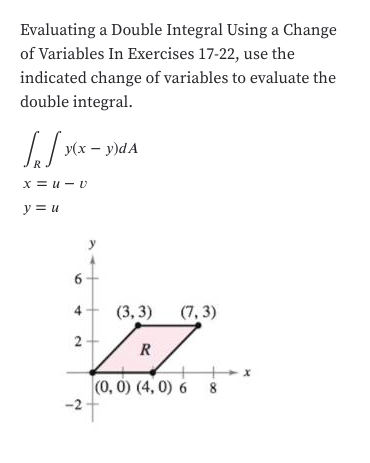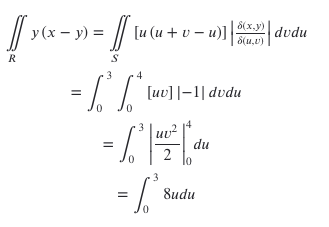# Evaluating a Double Integral Using a Changeof Variables In Exercises 17-22, use theindicated change of variables to evaluate thedouble integralУ(х — у)dAх%3D и — иy u64(3, 3)(7, 3)2x(0, 0) (4, 0) 6 8 y (x- y)lu (u + v- u)] |;S(x.y)S(u.v)dvduRue]- dedu003du2.=8udu

Question

I get how to do the problem up until ∫∫[u(u+v-u)]

where did they get [u(u+v-u)]  from? Please explainhelp_outlineImage TranscriptioncloseEvaluating a Double Integral Using a Change of Variables In Exercises 17-22, use the indicated change of variables to evaluate the double integral У(х — у)dA х%3D и — и y u 6 4 (3, 3) (7, 3) 2 x (0, 0) (4, 0) 6 8 fullscreenhelp_outlineImage Transcriptionclosey (x- y)lu (u + v- u)] |; S(x.y) S(u.v) dvdu R ue]- dedu 0 0 3 du 2. =8udu fullscreen
check_circleExpert Solution
Step 1

To determine the double integral using a suitable change of coordinates

Step 2

The region in x,y coordinates (bounded by the red lines)

Step 3

Consider the map x=u-v,y=v  (SEE correction). This is equivalent to u=x-y, v=y. The points O,A, B, C get transformed to O,P,Q, R as shown in the graph. For examplel...

### Want to see the full answer?

See Solution

#### Want to see this answer and more?

Solutions are written by subject experts who are available 24/7. Questions are typically answered within 1 hour*

See Solution
*Response times may vary by subject and question
Tagged in

### Math